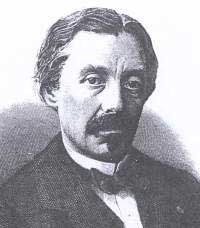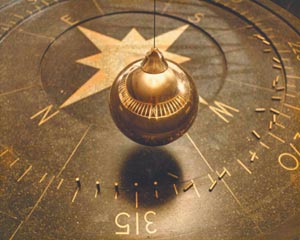Leon FoucaultUX25 / Sun conjunction

>>
Jean Bernard Léon Foucault (18 September 1819  11 February 1868) was a French physicist best known for the invention of the Foucault pendulum, a device demonstrating the effect of the Earth's rotation. He also made an early measurement of the speed of light, discovered eddy currents, and although he didn't invent it, is credited with naming the gyroscope. The Foucault crater on the Moon is named after him.
Foucault was the son of a publisher in Paris, where he was born on September 18, 1819.
<<
http://en.wikipedia.org/wiki/L%C3%A9on_FoucaultAssuming noon, 11:50:40 UT according to Astrodienst
Using RIYAL 3.13

Astrological Setting (Tropical - Placidus)

RIYAL Sat September 18 1819 UT 11h50m40s Lat48n52 Lon2e20 RADIX

CY118 = 0,05 Tau r                     Eris = 13,21 Aqu r
Nessus = 1,41 Tau r                   TD10 = 13,35 Lib
Ixion = 2,18 Ari r                         Chaos = 14,03 Sco
(Ascend) = 2,54 Sag                 Okyrhoe = 17,17 Sco
Teharonhi= 3,28 Can                 Radamantu= 17,31 Sag
Apogee = 4,14 Leo                   Amycus = 17,57 Ari r
Pholus = 4,20 Sco                     Bienor = 18,03 Gem
Pelion = 4,33 Aqu r                   Venus = 19,03 Vir
Cyllarus = 4,51 Sco                   Logos = 20,33 Cap r
Thereus = 5,36 Pis r                  Uranus = 20,40 Sag
Varuna = 6,01 Sco                    CZ118 = 21,07 Gem r
Ceto = 6,26 Pis r                       Sedna = 21,31 Pis r
Asbolus = 6,29 Leo                   Elatus = 22,23 Ari r
Mars = 7,20 Can                       Deucalion= 23,50 Pis r
Jupiter = 7,45 Aqu r                 Chariklo = 24,02 Lib
Typhon = 8,01 Cap r                 Quaoar = 24,30 Ari r
Mercury = 8,18 Vir                   Sun = 24,42 Vir
Pylenor = 8,48 Leo                    Hylonome = 24,42 Ari r
Crantor = 10,15 Leo                  Neptune = 25,57 Sag
Node = 11,11 Ari r                     (Midheav) = 26,15 Vir
Echeclus = 11,52 Leo               Orcus = 26,24 Lib
Huya = 11,52 Pis r                     Pluto = 26,35 Pis r
TL66 = 12,34 Sag                     Chiron = 27,02 Pis r
(Moon) = 13,01 Vir                    Saturn = 27,32 Pis r
_____________________________________________

Focused Minor Planets

UX25 = 24Vi49
Sun = 24Vi42
Pluto = 26Pi35 r
Deucalion= 23Pi50 r

Neptune = 25Sa57       Square
TC302 = 25Sa51

OR10 = 25Ca16            Sextile
Quaoar = 24Ar30 r       Quincunx
Hylonome = 24Ar42 r
Chariklo = 24Li02          Semisextile
______________________

Makemake = 8Cp59 r
Typhon = 8Cp01 r
Mercury = 8Vi18           Trine
AZ84 = 8Li10                 Square
______________________

GV9 = 19Aq34 r
Venus = 19Vi03            Quincunx
RM43 = 18Sa06            Sextile
______________________

Varuna = 6Sc01
Mars = 7Ca20 Trine
Jupiter = 7Aq45 r          T Square
TX300 = 7Le48
Asbolus = 6Le29
______________________

Orcus = 26Li24
Saturn = 27Pi32 r         Quincunx
Pluto = 26Pi35 r
Chiron = 27Pi02 r
OR10 = 25Ca16           Square
_______________________________________________

Posted to Centaurs (YahooGroups) on July 08, 2010

__________________________________________________________
__________________________________________________________